# Mass and Weight

## Mass

Mass is defined as the quantity of matter in a substance.

- The mass of an object is the same everywhere.

The unit of mass is kilogram [kg].

#### Instrument for measuring mass is:

Beam balance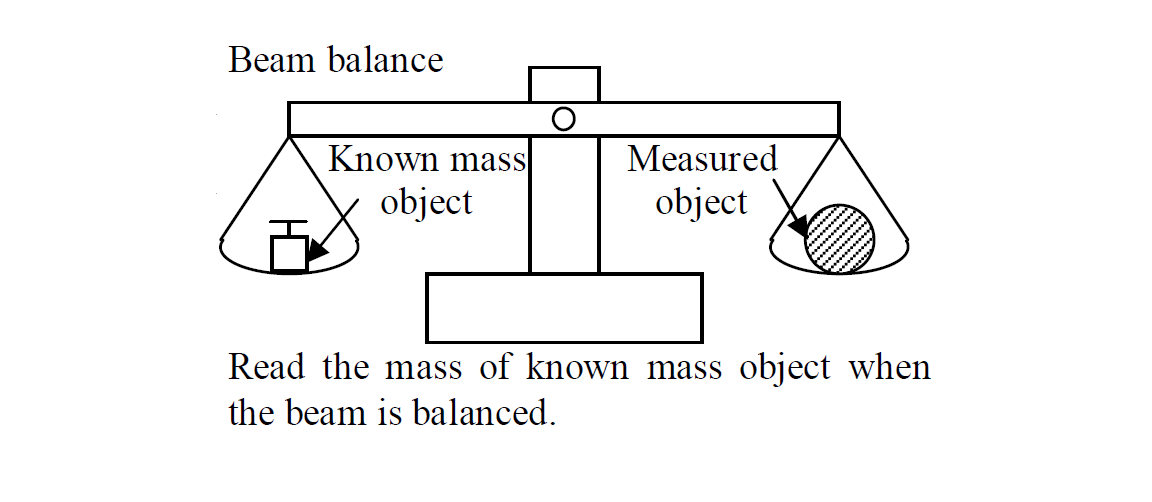## Weight

Weight is defined as the attractive force exerted on an object by gravity

- The weight of an object varies from place to place. (e.g. from the earth to the moon)

The unit of weight is Newton [N].

#### Instrument for measuring weight

Spring balance

Bathroom scale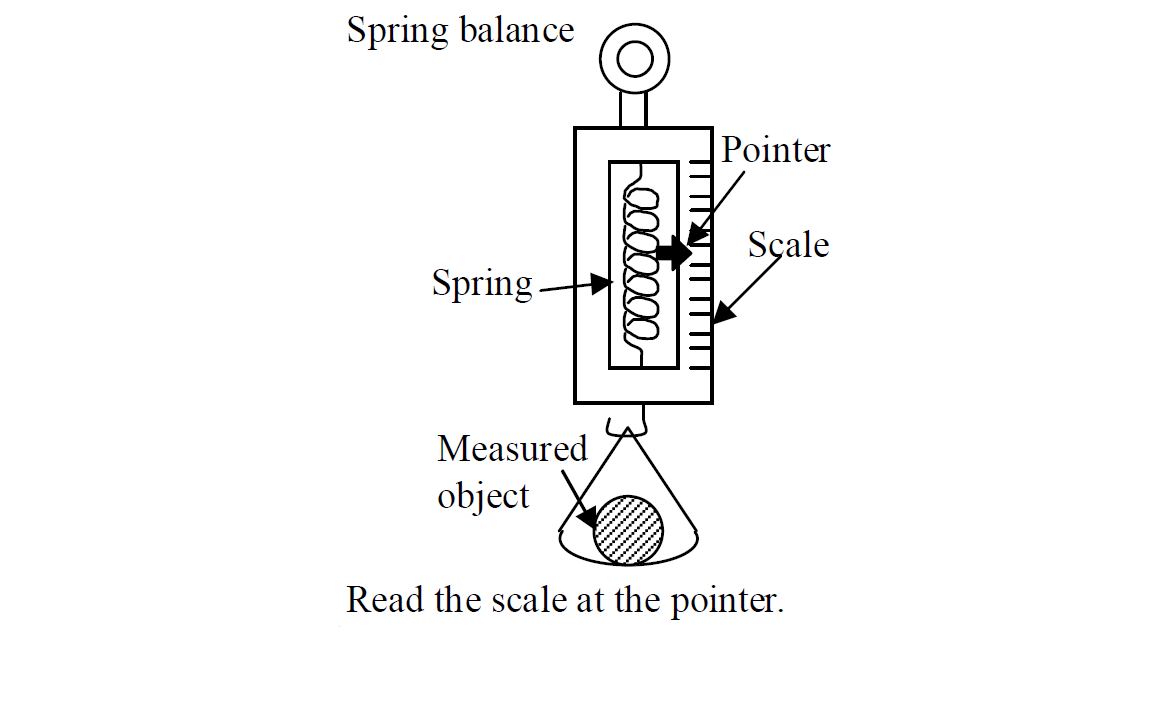#### Relationship between mass and weight

The weight of an object is directly proportional to its mass.

##### Formula

$W=mg$

w: weight [N]

m: mass [kg]

g: acceleration due to gravity[m/s2]

- Gravity on earth is 10m/s2

- Gravity of the moon is only about 1/6 that of the earth.

g = 10×16m/s2 (≈ 1.67 m/s2) on the moon

- No gravity in outer space.

g = 0 m/s2 in the outer space

The following table shows the changes to the mass and weight of an astronaut when he travels from the earth to the moon in a spacecraft.

$\begin{array}{|llll|}\hline & Earth& Moon& Outer Space\\ Mass& 60kg& 60kg& 60kg\\ Weight& 600N& 100N& 0N\\ \hline\end{array}$

The mass of a man is 70kg. What is his weight on the earth?

Solution

given that

m = 70kg

g = 10m/s2

W = ?

$W=mg$

$W=70×10$

$W=700N$

Answer: $W=700N$

#### Centre of gravity

The center of gravity of a body is defined as the point through which its whole weight appears to act.

below is the diagram that illustrate the center of gravity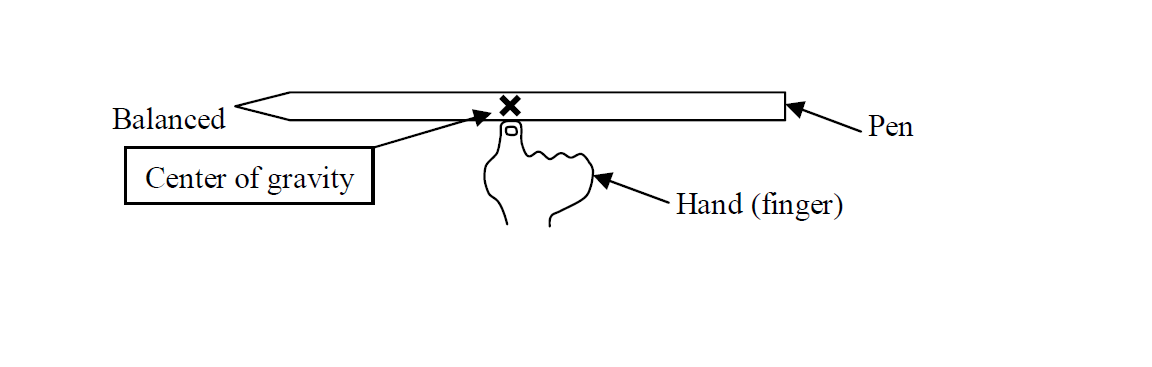#### Stability

The stability of an object is defined as the ability of an object to regain its original position after it has been displaced slightly.

The position of centre of gravity affects the stability of a body.

##### Example 1

Which is more stable? from the objects below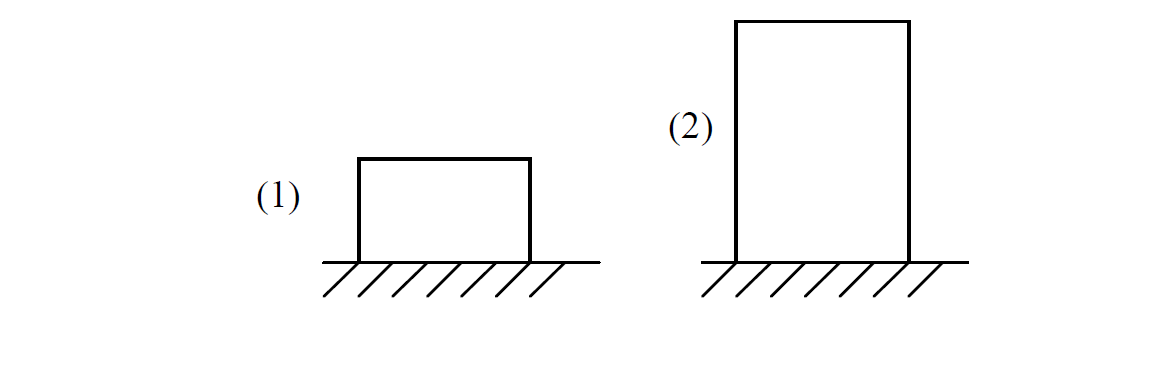(1) is more stable than (2) because it has a lower centre of gravity.

##### Example 2

Which is more stable? from the objects below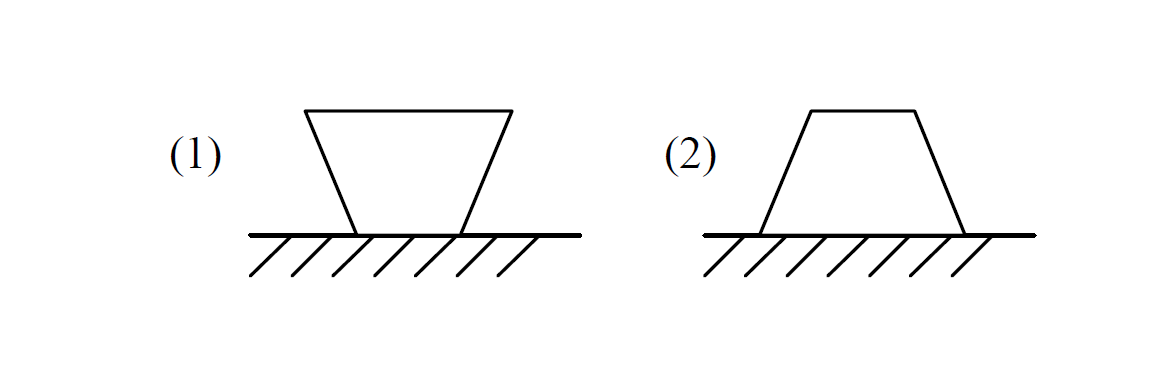(2) is more stable than (1) because it has a larger base area.

##### To increase the stability of an object,

the centre of gravity should be as low as possible.

the base area should be as large as possible.